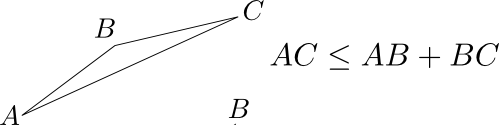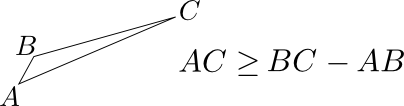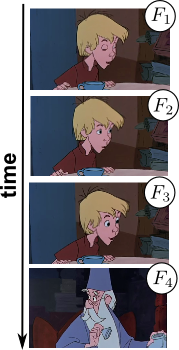# An Algorithm to Extract Looping GIFs From Videos

Yet another big problem of the Internet era tackled by Mathematics.

Looping GIFs are a very popular form of art on the Web, with two dedicated forums on Reddit (r/perfectloops and r/cinemagraphs) and countless Tumblr pages.Finding and extracting well-looping segments from a movie requires much attention and patience, and will likely leave you like this in front of your computer:To make things easier I wrote a Python script which automates the task. This post explains the math behind the algorithm and provides a few examples of use.

## When is a video segment well-looping ?

We will say that a video segment loops well when its first and last video frames are very similar. A video frame $F$ can be represented by a sequence of $N$ integers $(F, \cdots, F[N])$ whose values indicate the colors of the image’s pixels. For instance, $F$ $F$ and $F$ give the Red, Green, Blue values of the first pixel, $F$, $F$, $F$ define the color of the second pixel, etc.

Given two frames $F_1$, $F_2$ of a same video, we define the difference between these frames as the sum of the differences between their color values:

We will consider that the two frames are similar when $d(F_1,F_2)$ is under some arbitrary threshold $T$.

For what follows, it is important to note that $d(F_1, F_2)$ defines a distance between the frames, and can be seen as a generalization of the geometrical distance between two points in a plane:As a consequence $d(F_1,F_2)$ has nice mathematical properties which we will use in the next section to speed up computations.

## Finding well-looping segments

In this section we want to find the times (start and end) of all the well-looping video segments of duration 3 seconds or less in a given video. A simple way to do this is to compare each frame of the movie with all the frames in the previous three seconds. When we find two similar frames (that is, whose distance in under some pre-defined threshold $T$), we add their corresponding times to our list.

The problem is that this method requires a huge number of frame comparisons (around ten millions in a standard video) which takes hours. So let us see a few tricks to makes computations faster.

Trick 1: use reduced versions of the frames. HD videos frames can have millions of pixels, so computing the distance between them will require millions of operations. When reduced to small (150-pixel-wide) thumbnails these frames are still detailed enough for our purpose, and their distance can be computed much faster (they also take less place in the RAM).

Trick 2: use triangular inequalities. With this very efficient trick we will be able to deduce whether two frames match, without having to compute their distance. Since $d(F_1, F_2)$ defines a mathematical distance between two frames, many results from classical geometry apply, and in particular the following inequalities on the lengths of a triangle:The first inequality tells us that if A is very close to B which in turn is very close to C, then A is also close to C. In terms of video frames, this becomes:

In practice we will use it as follows: if we already know that a frame $F_1$ is very similar to a frame $F_2$, and that $F_2$ is very similar to another frame $F_3$, then we do not need to compute $d(F_1,F_3)$ to know that $F_1$ and $F_3$ are also very similar.

The second inequality tells us that if a point A is very near from B, and B is far from C, then A is also far from C. Or in terms of frames:

If $F_1$ is very similar to $F_2$, and $F_2$ is different from $F_3$, then we do not need to compute $d(F_1,F_3)$ to know that $F_1$ and $F_3$ are also very different.

Now it gets a little more complicated: we will apply these triangular inequalities to get information on the upper and lower bounds of the distances between frames, which will be updated every time we compute a distance between two frames. For instance, after computing the distance $d(F_1, F_2)$, the upper and lower bounds of $d(F_1, F_3)$, denoted $\overline{F_1F_3}$ and $\underline{F_1F_3}$, can be updated as follows:

If after the update we have $% $, we conclude that $F_1$ and $F_3$ are a good match. And if at some point $\underline{F_1F_3}>T$, we know that $F_1$ and $F_3$ don’t match. If we cannot decide whether $F_1$ and $F_3$ match using this technique, we will eventually need to compute $d(F_1, F_3)$, but then knowing $d(F_1, F_3)$ will in turn enable us to update the bounds on another distance, $d(F_1, F_4)$, and so on.

As an illustration, suppose that a video has the following frames in this order:When the algorithm arrives at $F_4$, it first computes the distance between this frame and $F_3$ and finds that they don’t match. At this point the algorithm has already found thaft $F_3$ is quite similar to $F_2$ and $F_1$, so it deduces that neither $F_1$ nor $F_2$ match with $F_4$ (and, certainly, neither do the dozen frames before ). In practice, this method avoids computing 80% to 90% of the distances between frames.

Trick 3: use an efficient formula for the distance. When we compute the distance between two frames using the formula from the last section, we need approximately $3N$ operations: $N$ subtractions, $N$ products, and $(N-1)$ additions to obtain the final sum. But the formula for $d(F_1, F_2)$ can also be rewritten under this form, known as the law of cosines:

where we used the following notations:

The interesting thing with this expression of $d(F_1, F_2)$ is that if we first compute the norm $\|F\|$ of each frame once, we can obtain the distance between any pair of $F_1$ and $F_2$ simply by computing $(F_1 \cdot F_2)$, which requires only $2N$ operations and is therefore 50% faster.

Another advantage of computing $\|F\|$ for each frame is that for two frames $F_1$ and $F_2$ we have

which provides initial values for the upper and lower bounds on the frame distances used in Trick 2:

Final algorithm in pseudo-code. Putting everything together, we obtain the following algorithm:

Here is the implementation in Python. The computation time may depend on the quality of the video file, but most movies I tried were processed in circa 20 minutes. Impressive, right, Eugene ?## Selecting interesting segments

The algorithm described in the previous section finds all pairs of matching frames, including consecutive frames (which often look very much alike) and frames from still segments (typically, black screens). So we end up with typically a hundred thousand video segments, only a few of which are really interesting, and we must find a way to filter out all the segments we don’t want before extracting GIFs. This filtering operation takes just a few seconds but its success depends greatly on the filtering criteria you use. Here are some examples that work well:

• The first and last frames must be separated by at least 0.5 second.
• There must be at least one frame in the sequence which doesn’t match at all with the first frame. This criterion enables to eliminate still segments.
• The start of the first frame must be at least 0.5 seconds after the start of the last extracted segment. This is to avoid duplicates (segments which start and end almost at the same times).

I try to be not too restrictive (to avoid filtering out good segments by accident) so I generally end up with about 200 GIFs, many of them them only midly interesting (blinking eyes and such). The last step is a manual filtering which looks like this:## Examples of use

I implemented this algorithm as a plugin of my Python video library MoviePy. Here is an example script with much details:

Here is what be obtain when we try it on Disney’s Snow White:

Some of these GIFs could be cut better, some are not really interesting (too short), and a few looping segments have been missed. I think the culprits are the parameters in the last filtering step, which could have been tuned better.

As another example, someone recently posted a Youtube video on r/perfectloops and required that it be transformed into a looping GIF. The following script does just that: it downloads the video from Youtube, finds the best times (t1,t2) to cut a looping sequence, and generates a GIF:And since you read until there, here is a more advanced trick for you: